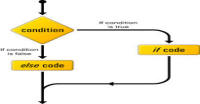# Basic Operators in Python

In this tutorials we will read about what operations can be perform in the python. You may consider operators as worker who perform some task.

### Types of Operator

Python language supports the following types of operators.

1. Arithmetic Operators
2. Assignment Operators
3. Comparison (Relational) Operators
4. Bitwise Operators
5. Logical Operators
6. Membership Operators
7. Identity Operators

Let us have a look on all operators one by one.

### 1) Arithmetic Operators:

Arithmetic operators are your maths operators. These are +, -, *, **, / , % and //.

Example:

a = 4 + 5 # + is an arithmetic operator

'**' is exponent operator. '//' operator returns the quotient after dividing the number. Example:

```>>> 2**3
8
>>> 3**2
9
>>> 5.0 // 2.0
2.0
>>> 5 // 2
2
```

Note: there is a difference between ‘/’ and ‘%’ operator. ‘/’ is divide operator while % is modulus operator. '/' performs different work in python 2 and python 3.

Python 2:

Example: 8 / 5 = 1 ( 8 = 5*1 + 3) while 8 % 5 = 3 ( 3 is reminder)

Python 3:

Example: 8 / 5 = 1.6 while 8 % 5 = 3 ( 3 is reminder)

### 2) Assignment Operators:

Assignment operator assigns values to variables. Assignment operators are:

OperatorDescriptionExample
=Simple we are giving a value to variablex = y + z
+=add y into x and give result to xx += y is equivalent to x = x + y
-=subtract y from x and give result to xx -= y is equivalent to x = x - y
*=multiply y with x and give result to xx *= y is equivalent to x = x * y
/=divide x by y and give result to xx /= y is equivalent to x = x / y
%=take modulo of x and give result to xx %= y is equivalent to x = x % y
**=rise y power of x and give result to xx **= y is equivalent to x = x ** y
//=floor division of x by y and give result to xx //= y is equivalent to x = x // y

Examples:

```>>> a = 4
>>> print(a)
4
>>> a += 5
>>> print(a)
9
>>> a -= 2
>>> print(a)
7
>>> a *= 6
>>> print(a)
42
>>> a /= 5
>>> print(a)
8.4
>>> b = 14
>>> b % 3
2
>>> b %= 3
>>> print(b)
2
>>> b **= 3
>>> print(b)
8
>>> b //= 5
>>> print(b)
1
```

NOTE: In python 2, you will get result equal to 8 instead of 8.4 in line 15.

### 3) Comparison Operators:

Comparison operators are used to compare one operand to another. These compares two operands and return true if comparison is right otherwise false.

 Operators Descriptors == both are equal != both are not equal < left is smaller > right is smaller <= left is smaller or equal >= right is smaller or equal <> similar to !=

### 3) Bitwise Operators:

Bitwise operators performs operations on individual bits in an integer. Bitwise operators are & (and), | (or), << (left sift) , >> (right sift), ^ (XOR) and ~ (NOR).

Example: 14 & 3 = 2 (00001110 & 00000011 = 00000010 these numbers are in binary).

### 4) Logical Operators:

Logical operators are similar to 'and' and 'or' logical gates. You can use 'or' and 'and' for these gates.

```>>> 2 and 2
2
>>> 2 and 3
3
>>> 2 and 0
0
>>> 0 and 3
0
>>> 2 or 3
2
>>> 3 or 2
3
>>> 0 or 2
2
>>> 2 or 0
2
```

### 5) Membership Operators:

There are two member operators 'in' and 'not in'. These are to check if a variable is present in a sequence, such as strings, lists, or tuples.

```>>> sequence = [2, 2.3, 'name', 'harish', 'number']
>>> 2 in sequence
True
>>> 3 in sequence
False
>>> a = 'string'
>>> 't' in a
True
>>> 'p' in a
False
>>> 'p' not in a
True
```

### 6) Identity Operators:

There are two identity operators 'is' and 'is not'. These are to check if both objects are identical or not.

```>>> a = 12
>>> b = 12
>>> a is b
True
>>> b is a
True
>>> a is 12
True
>>> b = 13
>>> a is b
False
>>> b is 13
True
>>> 12 is 12
True
>>> 12 is not 13
True
```

In the next tutorial we will read about Decision Making Statements, i.e., if and if else statements.

#### Click on banner below to Learn: PHP with Laravel for beginners - Become a Master in LaravelHarish, a technical core team member at www.lyflink.com with five year experience in full stack web and mobile development, spends most of his time on coding, reading, analysing and curiously following businesses environments. He is a non-graduate alumni from IIT Roorkee, Computer Science and frequently writes on both technical and business topics.

### Related ArticlesPHP stands for PHP: Hypertext Preprocessor. PHP is known as a server side scripting and interpreter language for web ...
##### Introduction to PHPIn this tutorial, we will study about conditional statements (if else statements and switch statements). These statem...
##### Conditional statements in phpIn this tutorial, we will study about strings and operations upon strings. Due to long length of this section, I have...

### The Complete Web Developer Course - Build 25 Websites